• #将有序数组转为二叉树并实现层序遍历(BFS) class BitNode: def __init__(self): self.data=None self.lchild=None self.rchild=None def arrtotree(self,arr,start,end): #将数组转为二叉树苏 root=None if ...
#将有序数组转为二叉树并实现层序遍历(BFS)
class BitNode:
def __init__(self):
self.data=None
self.lchild=None
self.rchild=None
def arrtotree(self,arr,start,end): #将数组转为二叉树苏
root=None
if end>=start:
root=BitNode()
mid=(start+end+1)//2 #将数组中间结点当做树的根节点
root.data=arr[mid]
root.lchild=BitNode().arrtotree(arr,start,mid-1) #递归构造左子树
root.rchild=BitNode().arrtotree(arr,mid+1,end) #递归构造右子树
else:
root=None
return root
def bfs(self,root): #广度优先遍历
from collections import deque
if root==None:
return
queue=deque()
queue.append(root) #树的根节点放入队列
while len(queue)>0:
p=queue.popleft() #访问当前节点
print(p.data)
if p.lchild!=None:
queue.append(p.lchild)
if p.rchild!=None:
queue.append(p.rchild)

if __name__=='__main__':
arr=[1,2,3,4,5,6,7,8,9,10]
root=BitNode().arrtotree(arr,0,(len(arr)-1))
BitNode().bfs(root)

展开全文python 数据结构
• 文章目录一、用数组存储层序遍历的堆1.按层序遍历2.算左子节点、算右子节点、算父节点的下标（1）伪代码结果（2）C++二、堆1.大顶堆和小顶堆2.堆的高度和层三、大顶堆排序算法1.MAX-HEAPIFY2.BUILD-MAX-HEAP3.堆排序...

一、用数组存储层序遍历的堆

1.按层序遍历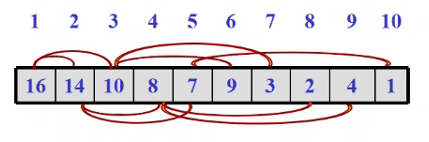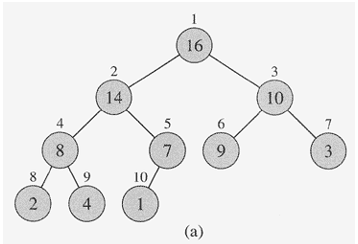2.算左子节点、算右子节点、算父节点的下标

（1）伪代码结果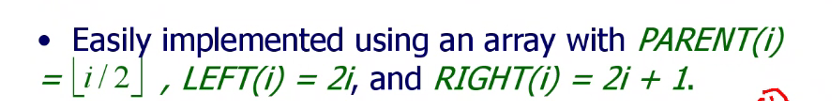i为本结点，j为所求结点，采用[1,n]

• LEFT(i) = 2 ∗ i \small{\text{LEFT(i)}}=2*i

• RIGHT(i) = 2 ∗ i + 1 \small{\text{RIGHT(i)}}=2*i+1

• PARENT(i) = ⌊ i / 2 ⌋ \small{\text{PARENT(i)}}=\lfloor i/2 \rfloor

（2）C++

i为本结点，j为所求结点，采用[0,n-1]

• LEFT(i)： j + 1 = 2 ( i + 1 ) ， 则 j = 2 ∗ i + 1 j+1=2(i+1)，则j=2*i+1

• RIGHT(i)： j + 1 = 2 ( i + 1 ) + 1 ， 则 j = 2 ( i + 1 ) j+1=2(i+1)+1，则j=2(i+1)

• PARENT(i)： j + 1 = ⌊ ( i + 1 ) / 2 ⌋ j+1=\lfloor (i+1)/2 \rfloor ，则 j = ⌊ ( i + 1 ) / 2 ⌋ − 1 j=\lfloor{(i+1)/2}\rfloor-1

二、堆

1.大顶堆和小顶堆

• 大顶堆：父结点比左右子节点的值都大
• 小顶堆：父结点比左右子节点的值都小

2.堆的高度和层

• 高度： l o g 2 n log_2{n}
• 层=高度+1

三、大顶堆排序算法

• MAX-HEAPIFY（调整本结点以下的顶堆为大顶堆）
• BUILD-MAX-HEAP（无序数组→大顶堆）

1.MAX-HEAPIFY

// 伪代码
// 数组A，要调整的本结点下标i（调整本结点以下的顶堆为大顶堆）
MAX-HEAPIFY(A, i)
// 获得左孩子下标
l ← LEFT(i)
// 获得右孩子下标
r ← RIGHT(i)

/* 比较本节点、左子节点、右子节点的值，让largest为最大下标 */

// 检验l是否没有越界，并且当左孩子的值比本结点的更大时
if l ≤ heap-size[A] and A[l] > A[i]
// 则最大值的下标largest为左孩子的下标l
then largest ← l
// 否则，当本结点的值比左孩子的更大时，则最大值的下标largest为本结点的下标i
else largest ← i

// 检验r是否没有越界，并且当右孩子的值比最大值结点的更大时
if r ≤ heap-size[A] and A[r] > A[largest]
// 则最大值的下标largest为右孩子的下标r
then largest ← r

// 当最大值的下标不是本结点i时（需要调整因交换而破坏的下一层大顶堆）时
if largest ≠ i
// 则交换本结点和最大值结点的值
then exchange A[i] ⇿ A[largest]
// 对最大值结点的大顶堆进行调整
MAX-HEAPIFY(A, largest)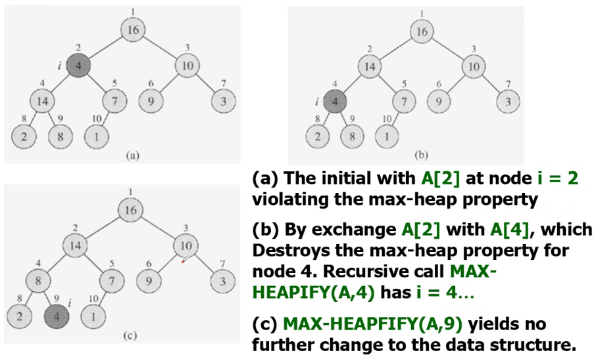2.BUILD-MAX-HEAP

感觉像是冒泡排序一样，大的元素逐渐浮上堆顶，小的元素沉积下去。

注意：完事后数组不是降序的，左右子节点的大小还没有排

// 伪代码
// 无序数组A（我们想要将无序数组→大顶堆）
BUILD-MAX-HEAP(A)
// 堆的大小是数组A的长度
heap-size[A] ← length[A]
// 从一半的下标开始（最后一个叶子节点的父结点下标，直接用PARENT(n)）
// 下标递减往上遍历各个结点。
for i ← ⌊ length[A] / 2 ⌋ downto 1
// 对每个结点进行MAX-HEAPIFY（调整本结点以下的顶堆为大顶堆）
do MAX-HEAPIFY(A, i)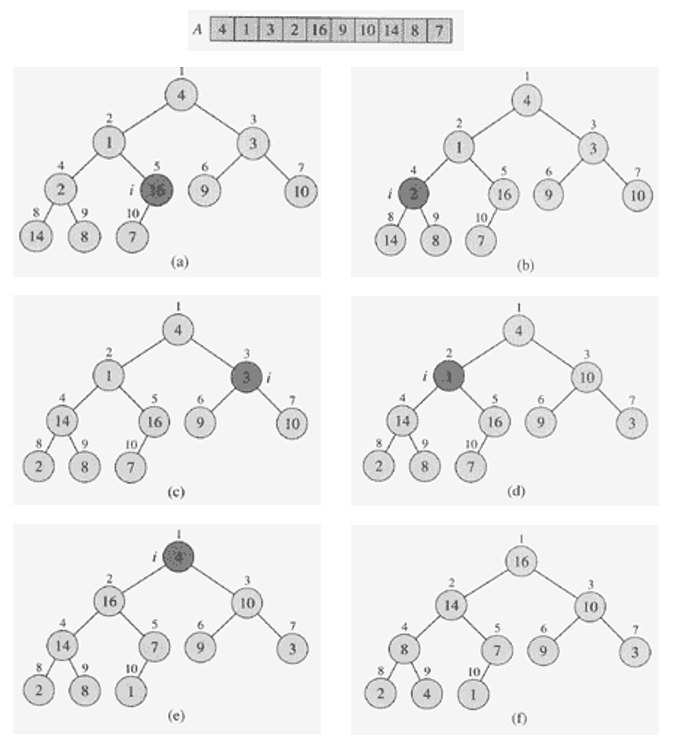PS：循环不变式做验证的过程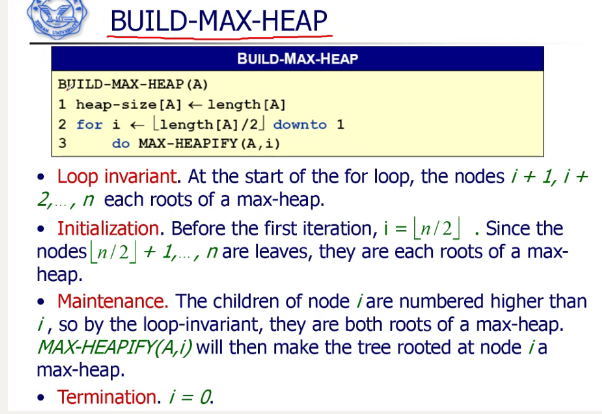3.堆排序算法

// 伪代码
// 无序数组A
HEAPSORT(A)
// 将无序数组→大顶堆
BUILD-MAX-HEAP(A)
// i表示每次循环中堆的最后一个叶子结点
// 直到只剩下一个根节点（因为不用MAX-HEAPIFY了）
for i ← length[A] downto 2
// 交换树根A和最后的叶子结点A[i]
do	exchange A ⇿ A[i]
// 剪掉这个叶子结点（堆中目前的最大值），即堆的大小减1
heap-size[A] ← heap-size[A] - 1
// 对树根进行MAX-HEAPIFY，因为交换了树根A和最后的叶子结点A[i]
MAX-HEAPIFY(A, 1)

数组：增序（因为是倒着减去堆中的最大值）

PS：A[i]是最后的叶子结点，而不是最小值的结点。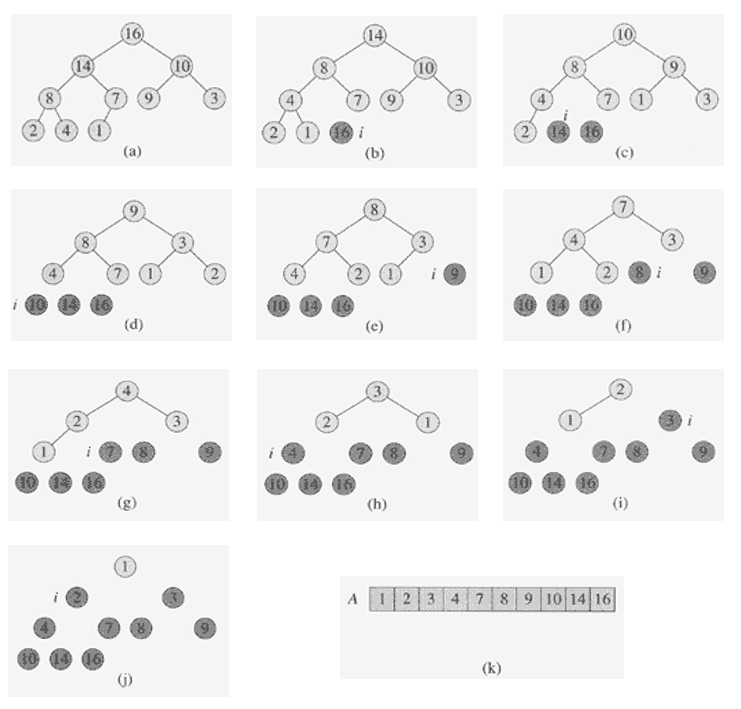4.时间复杂度

BUILD-MAX-HEAP n l g n nlgn

堆排序算法 n l g n nlgn

共：还是 n l g n nlgn

四、C++实现

#include <iostream>

using namespace std;

// 初始化于BUILD_MAX_HEAP()，用于MAX_HEAPIFY()中
int heap_size;

int LEFT(int i)
{
// 伪代码是2 * i
return 2 * i + 1;
}

int RIGHT(int i)
{
// 伪代码是2 * i + 1
return 2 * (i + 1);
}

int PARENT(int i)
{
// 伪代码是PARENT(i)=⌊i/2⌋
return (int)((i + 1) / 2) - 1;
// 或者 return floor((i + 1) / 2) - 1; 需要 #include <math.h> // for floor()
}

// 调整本结点以下的顶堆为大顶堆
void MAX_HEAPIFY(int A[], int i)
{
// 获得左孩子下标
int l = LEFT(i);
// 获得右孩子下标
int r = RIGHT(i);

/* 比较本节点、左子节点、右子节点的值，让largest为最大下标 */

// 本结点、左孩子、右孩子中值最大的结点下标。先假设本结点最大
int largest = i;
// 检验l是否没有越界，并且当左孩子的值比本结点的更大时
if (l < heap_size && A[l] > A[i])
{
// 则最大值的下标largest为左孩子的下标l
largest = l;
}

// 检验r是否没有越界，并且当右孩子的值比最大值结点的更大时
if (r < heap_size && A[r] > A[largest])
{
// 则最大值的下标largest为右孩子的下标r
largest = r;
}

// 当最大值的下标不是本结点i时（需要调整因交换而破坏的下一层大顶堆）时
if (largest != i)
{
// 则交换本结点A[i]和最大值结点的值A[largest]
swap(A[i], A[largest]);
// 对最大值结点的大顶堆进行调整
MAX_HEAPIFY(A, largest);
}
}

// 无序数组→大顶堆和初始化heap_size
void BUILD_MAX_HEAP(int A[], int length)
{
// 初始化全局变量heap_size，为数组A的元素个数
heap_size = length;
// 从一半的下标开始（最后一个叶子节点的父结点下标，直接用PARENT(heap_size -1)）
// 下标递减往上遍历各个结点。
for (int i = PARENT(heap_size - 1); i >= 0; i--)
{
// 对每个结点进行MAX-HEAPIFY（调整本结点以下的顶堆为大顶堆）
MAX_HEAPIFY(A, i);
}
}

// 要调用的堆排序函数
void HEAPSORT(int A[], int length)
{
// 无序数组→大顶堆和初始化heap_size
BUILD_MAX_HEAP(A, length);
// i表示每次循环中堆的最后一个叶子结点
// 直到只剩下一个根节点（下标0）（因为不用MAX-HEAPIFY了）
for (int i = heap_size - 1; i >= 1; i--)
{
// 交换树根A和最后的叶子结点A[i]
swap(A, A[i]);
// 剪掉这个叶子结点（堆中目前的最大值），即堆的大小减1
heap_size--;
// 对树根进行MAX-HEAPIFY，因为交换了树根A和最后的叶子结点A[i]
MAX_HEAPIFY(A, 0);
}
}

int main()
{
int A[] = {16, 14, 10, 8, 7, 9, 3, 2, 4, 1};
int length = sizeof(A) / sizeof(A);
HEAPSORT(A, length);
for (int i = 0; i < length; i++)
{
cout << A[i] << " ";
}
cout << endl;
return 0;
}

五、扩展：优先级队列

手写优先级队列

展开全文• 给定一个二叉树层序遍历（只要该层有结点，那么该层的空结点也要访问）的整型数据数组数组中的0代表该结点为NULL，要求你根据该数组输出该二叉树先序遍历的结点值，C++代码实现如下： #include<iostream> ...

给定一个二叉树层序遍历（只要该层有结点，那么该层的空结点也要访问）的整型数据数组，数组中的0代表该结点为NULL，要求你根据该数组输出该二叉树先序遍历的结点值，C++代码实现如下：

#include<iostream>
using namespace std;

//关键在于对数组下标的处理
//从0开始存储，那么第i个结点的左孩子就是2*i+1，右孩子就是2*i+2。
//如果从1开始存储，那么第i个结点的左孩子是2*i，右孩子是2*i+1。
//因为数组是从0开始存储的，所以i从0开始往右找它的子结点
void preShow(int *arr,bool *visited,int i,int n){
//这个函数类似于二叉树的先序遍历
if(i>=n)
return;
if(visited[i]){
cout<<arr[i]<<" ";
}
//一直往左走，走到尽头了再走右边
preShow(arr,visited,2*i+1,n);
preShow(arr,visited,2*i+2,n);
}

void preOrder(int *arr,int n){
//c++中bool的默认值是不确定的
bool* visited=new bool[n];
for(int i=0;i<n;i++){
visited[i]= false;
}
//核心思路是将0转化为类似NULL的标识，这里用布尔值也行
for(int i=0;i<n;i++){
if(arr[i]>0){
visited[i]=true;
}
}
preShow(arr,visited,0,n);
cout<<endl;
}

int main(){
int t;
cin>>t;
//t含义是进行t次的循环，每一次循环处理一个数组
for(int i=0;i<t;i++){
//n是指该数组的大小
int n;
cin>>n;
//arr就是储存层序遍历数据的数组
int *arr=new int[n];
for(int j=0;j<n;j++){
cin>>arr[j];
}
//一个函数处理好题目要求
preOrder(arr,n);
}
return 0;
}

我是花花，祝自己也祝您变强了~

展开全文数据结构 c++
• 0、这是最基本的层序遍历算法，没有... * 层序遍历,使用了ArrayDeque，一个循环数组，性能很好 * @param root */ public void sequenceSearch(Node root){ checkRoot(root); Queue fkQueue = new ArrayDeque();
• 二叉树的前中后序遍历（递归实现） #include <iostream> #include <cstdio> #include <string> #include <vector> #include <queue> #include <stack> #include <algorithm&...

结构体数组存储 递归实现

#include <iostream>
#include <cstdio>
#include <string>
#include <vector>
#include <queue>
#include <stack>
#include <algorithm>
using namespace std;
typedef long long ll;
#define maxn 1000005
#define mod 7654321
#define NIL -1

struct Tree
{
int parent,left,right;
}T[maxn];

//前序遍历(Traversal)
void PreTraversal(int u)
{
if(u==NIL)
return;
cout<<" "<<u;
PreTraversal(T[u].left);
PreTraversal(T[u].right);
}

//中序遍历
void InTraversal(int u)
{
if(u==NIL)
return;
InTraversal(T[u].left);
cout<<" "<<u;
InTraversal(T[u].right);
}

//后序遍历
void PostTraversal(int u)
{
if(u==NIL)
return;
PostTraversal(T[u].left);
PostTraversal(T[u].right);
cout<<" "<<u;
}

//层序遍历
void SequenceTraversal(int u)
{
queue<int> q;
q.push(u);
while(!q.empty())
{
int temp=q.front();
cout<<" "<<temp;
if(T[temp].left!=NIL)
q.push(T[temp].left);
if(T[temp].right!=NIL)
q.push(T[temp].right);
q.pop();
}
}

int main()
{
int value,l,r,root=0;
int n;
cin>>n;

//父节点的初始化
for(int i=0;i<n;i++) T[i].parent=NIL;

//结构体数组存储
for(int i=0;i<n;i++)
{
cin>>value>>l>>r;
T[value].left=l;
T[value].right=r;
if(l!=NIL) T[l].parent=value;
if(r!=NIL) T[r].parent=value;
}

//查找根节点
for(int i=0;i<n;i++)
if(T[i].parent==NIL)
root=i;

cout<<"PreTraversal"<<endl;
PreTraversal(root);cout<<endl;

cout<<"InTraversal"<<endl;
InTraversal(root);cout<<endl;

cout<<"PostTraversal"<<endl;
PostTraversal(root);cout<<endl;

cout<<"SequenceTraversal"<<endl;
SequenceTraversal(root);cout<<endl;

return 0;
}

展开全文• 1、思路根节点存储数组的第i个值，则其左右节点分别存储数组的第2i+1和第2i+2个值2、代码import java.util.*;class TreeNode{int val;TreeNode left;TreeNode right;public TreeNode(int x){val = x;}}public class ...
• 用代码实现数组以树的方式进行前序遍历 /** * 数组存储顺序二叉树（把数组用树前序遍历方式输出） * * 1.树的左子节点为 父节点坐标*2+1 * 2.树的右子节点为 父节点坐标*2+2 * 3. 父节点坐标 = （当前节点-1）/...
• void solve(int ALeft, int ARight, int TRoot) ... // TRoot是结果树序列的数组下标 n = ARight - ALeft + 1; if (n==0) return; L = GetLeftLength(n); // 计算出n个结点的树其左子树有多少个结点 T[T...
• 基于数组实现的树遍历方法

千次阅读 2021-03-06 13:28:45
即按照左子树 满二叉树常常用数组来存储 ## 基于数组实现的树遍历方法 如下图所示二叉树，若讲其存在数组中，其并不是该树所展示的样子，并且也并非有序的，不过由于BST的性质可知，其中序遍历即有序的。...算法 数据结构 二叉树
• 下面的实现为了简单，树的实现用了全局的数组遍量实现。 #include #include #include using namespace std; #define MaxTree 10 #define ElementType char #define Tree int #define Null -1 struct ...
• 二叉树的层序遍历（两种方法实现）

万次阅读 多人点赞 2018-06-24 23:51:59
两种方法实现二叉树的层序遍历 1、说明 二叉树的层序遍历是面试经常会被考察的知识点，甚至要求当场写出实现过程。 层序遍历所要解决的问题很好理解，就是按二叉树从上到下，从左到右依次打印每个节点中存储的...
• 2.层序遍历为广度优先遍历，需要队列实现。 思路方法： 1.首先需要依靠一个队列 2.先把头节点放进去，然后当队列不为空的时候弹出元素，并且记录当前弹出的元素 3.判断弹出的元素的左孩子为不为空，不是空，那么入...队列 二叉树 java 数据结构
• p154 紫书原文： 纠正一下这里的newnode()函数...树的层序遍历数组(静态链表)实现: #include<cstdio> #include<cstring> #include<vector> #include<queue> using namespace std; const int二叉树 算法 c++
• 层次遍历所有的结点 # 层次遍历所有的结点 def BFS(root): queue, result = [root], [] while queue: node = queue.pop(0) result.append(node.value) if node.left: queue.append(node.left) if node.right: queue....二叉树 遍历 层次遍历 数组 创建二叉树
• 二叉树层序遍历（C语言）

千次阅读 多人点赞 2021-04-25 22:14:28
二叉树的层序遍历即从上到下，在每一层从左...自然，本题还可以用数组实现。 代码： #include <stdio.h> #include <stdlib.h> #define QueueMax 100 typedef struct Node { char data; struct Node *二叉树 队列 数据结构
• 两种方法实现二叉树的层序遍历 1、说明 二叉树的层...
• 要求是将层序遍历的结果，第一层中节点的值域存在二维数组的第一个vector中，第二层的存在第二个中。依次继续。 创建一个二维数组作为最终输出结果，创建一个队列用来取每层的拿到的值域，创建一个vector对象...c++
• 二叉树的层序遍历：一般基于队列的实现 首先将二叉树的根节点push到队列中。 判断队列不为空就输出队头元素。 判断当前对头节点是否有孩子节点，有则push到队列中。 循环操作，直到队列为空。 代码如下： void...
• #include <stdio.h> #include <stdlib.h> #define MAX 100 struct treeNode { char data; struct treeNode* LChild; struct treeNode* RChild; }; struct treeNode* createNode(char data)... struct...
• 实际上就是广度优先遍历, 借助一个队列（这里用数组代替）就可以实现: 1、先将root节点加入队列 2、队列不为空时取队列首节点 3、打印节点的值，然后将该节点的左、右子节点先后加入队尾（核心步骤，广度优先体现在...python实现 数据结构 二叉树 广度优先遍历
• 二叉树的层序遍历 给你一个二叉树，请你返回其按 层序遍历 得到的节点值。 （即逐层地，从左到右访问所有节点）。 示例： 二叉树：[3,9,20,null,null,15,7], 3 / \ 9 20 / \ 15 7 返回其层次遍历结果...二叉树
• 给你一个二叉树，请你返回其按 层序遍历 得到的节点值。 （即逐层地，从左到右访问所有节点）。 示例： 二叉树：[3,9,20,null,null,15,7], 3 / \ 9 20 / \ 15 7 返回其层次遍历结果： [ , [9,20], ...层序遍历 深度遍历 二叉树的层序遍历
• 层序遍历要求输出的是一个二维数组，而bfs的遍历结果是一维数组，无法区分每一层。 那么我们如何给bfs的遍历结果分层，这里用到一个辅助空间，队列。 我们先把根节点放到队列中，然后不断遍历队列。 根节点是树...二叉树
• 三行代码递归实现二叉树层序遍历

千次阅读 多人点赞 2018-02-06 13:31:51
二叉树的层序遍历网上大部分都是使用队列的出队和入队来实现的,这次我用三行代码递归实现二叉树的层序遍历. 层序 下图是一个简单的二叉树,层序就是一行一行的往下读取,这个二叉树的层序结果便是: 1234567 ...二叉树 递归
• 1 题目 给你一个二叉树，请你返回其按 层序...这道题的主要思路是用队列来进行层序遍历，由于需要区分每一层，因此额外使用一个数组来存储下一层的节点 3代码 /** * Definition for a binary tree node. * function
• /** * Definition for a binary tree node. * struct TreeNode { * int val; * TreeNode *left; * TreeNode *right; * TreeNode(int x) : val(x), left(NULL), right(NULL) {} * };...clas...数据结构 算法
• 二叉树的层序遍历---java实现

千次阅读 2019-07-17 21:24:12
1、二叉树的层序遍历 即按照层输出节点 1.2 按照之字型打印 即奇数行从左往右打印，偶数行从右往左打印。 那么可以借助栈先进后出的特点实现之字形打印二叉树，设两个栈 stack1，stack2，stack2为辅助栈 ...二叉树的层序遍历 层序遍历 二叉树 数据结构
• 过队列的方式实现层序遍历，层序遍历与 BFS的不同点在于，层序遍历返回的每一层是一个list，而BFS是所有层拼起来的一个数组 class Solution(object): def levelorderTraversal(self, root: TreeNode): if root ...数据结构与算法 leetcode 二叉树 二叉树层序遍历
• #include #include/*** 二叉树二叉链表之非递归遍历：层序遍历* 算法思想：借助一个队列；根树进队；队不为空时循环“从队列中出一个树p，访问该树根结点；* 若它有左子树，左子树进队；若它有右子树，右子树进队。...c语言二叉树层序遍历
• 数据结构实验之二叉树五：层序遍历 Time Limit:1000 msMemory Limit:65536 KiB SubmitStatistic Problem Description 已知一个按先序输入的字符序列，如abd,,eg,,,cf,,,(其中,表示空结点)。请建立二叉树并求......